StatLect

# Covariance stationary

A sequence of random variables is covariance stationary if:

• all the terms of the sequence have the same mean;

• the covariance between any two terms of the sequence depends only on the relative position of the two terms and not on their absolute position.

By relative position of two terms we mean how far apart they are located from each other in the sequence.

Instead, the absolute position refers to where they are located in the sequence.## Synonyms

Covariance stationary sequences are also called:

• weakly stationary sequences;

• covariance stationary processes;

• weakly stationary processes.

## Definition

This is a formal definition.

Definition A sequence of random variablesis covariance stationary if and only if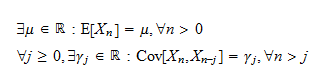In words:

1. all the terms of the sequence have mean;

2. the covariancedepends only on the relative positionand not on the absolute position.

Note that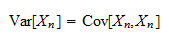which implies that a weakly stationary process has constant variance.

## Definition for random vectors

The definition above applies without modifications to sequences of random vectors.

In the case of a sequence of vectors,is a vector of expected values andis a matrix of covariances between the entries of the two vectorsand(a cross-covariance matrix).

## Examples

Let us make some examples.

### Example 1 - White noise

The simplest example is the so called white noise process, a sequencethat satisfies the following three conditions for anyand:whereis a positive constant.

### Example 2 - Autoregressive process

Letbe the white noise process of the previous example.

A first-order autoregressive process is a sequencewhose terms satisfywhereis a constant and the recursion starts from a random variableuncorrelated with the terms of.

The expected values of the terms of the sequence are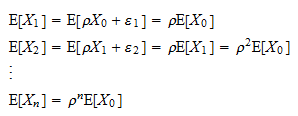For the processto be weakly stationary, the first condition that needs to be satisfied is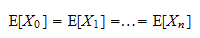which is satisfied only if.

The variances are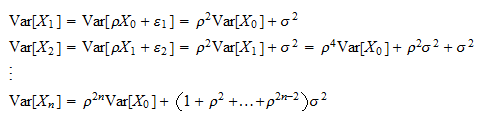The variances remain finite asgrows only if. Furthermore, the condition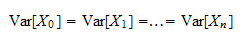is satisfied only ifwhich can be shown, for example, by solving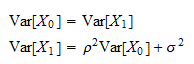As proved in the lecture on autocorrelation, the covariance between any two terms of the sequence iswhich satisfies the condition stated in the definition of weak stationarity (the covariance depends only on).

Thus, the sequenceis covariance stationary only if## Weakly vs strictly stationary

A stronger concept of stationarity is that of strict stationarity.

A sequenceis said to be strictly stationary if and only ifand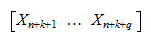have the same joint distribution for any,and.

## More details

Other concepts related to covariance stationarity can be found in the lecture on sequences of random variables.

Previous entry: Covariance formula

Next entry: Critical value